# albatross¶A framework for statistical modelling in C++, with a focus on Gaussian processes.

## Features¶

• Gaussian Process Regression using composable covariance functions and works with custom data types.
• Bayesian inferrence using an ensemble MCMC sampler based off emcee.
• Helpful utilities for working with datasets using the split apply combine approach.
• An interface around nlopt to make model tuning straight forward.
• Evaluation utilities, with a focus on cross validation.
• Parameter handling which makes it easy to get and set parameters in a standardized way and (de)serialize models.

## Why Albatross?¶

First let’s start with a bigger question, “Why write anything from scratch when you can use external packages?”. Opinions may vary, but some reasonable answers are:

• The external packages don’t quite do what you want.
• You think you can do it better.
• You don’t want any dependencies.

In on our situation it’s mostly the first; we want to build statistical models in a way that accommodates the research phase of development (rapid model iteration, evaluation, comparison, and tuning) but also runs fast in a production environment. So while just about everything in albatross could also be done with python packages such as pandas, scikit-learn, emcee and george, using them directly just wasn’t practical. Instead we started developing albatross, which draws heavily on paradigms we liked from those packages but with an emphasis on compile time safety and speed. In short you could say albatross is:

“A package containing some of the stats modeling tools from python that are missing in C++”

## Install¶

albatross is a header only library so incorporating it in your C++ project should be as simple as adding ./albatross as an include directory.

### Install as a submodule¶

If you’re using git you can run git submodule add https://github.com/swift-nav/albatross.git)

Then make sure you’ve run git submodule update --recursive --init to be sure all the third party libraries required by albatross are also up to date.

### Tests and Examples¶

If you want to run the tests you can do so using cmake,

mkdir build;
cd build;
cmake ../
make run_albatross_unit_tests


Similarly you can make and run the examples,

make sinc_example
./examples/sinc_example -input ./examples/sinc_input.csv -output ./examples/sinc_predictions.csv


and plot the results (though this’ll require a numerical python environment),

python ../examples/plot_example_predictions.py ./examples/sinc_input.csv ./examples/sinc_predictions.csv


#### 1D Example¶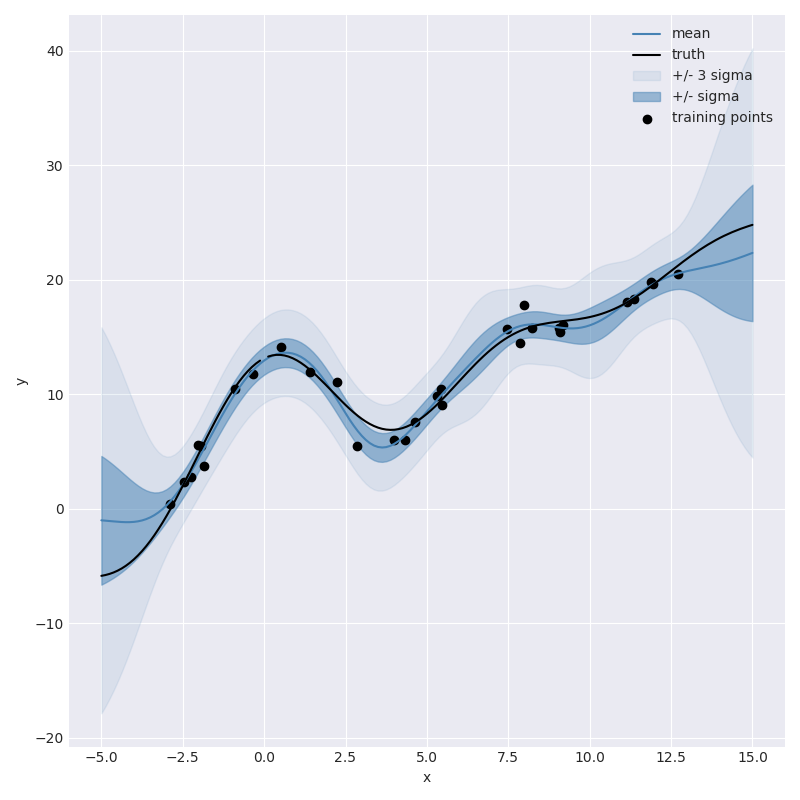##### Introduction¶

Regression problems typically involve estimating some unknown function $$f$$ from a set of noisy data $$y$$. As an example we can attempt to estimate a function $$f(x)$$,

$f(x) = a x + b + \mbox{something_nonlinear}(x)$

based off of noisy observations,

$y = f(x) + \mathcal{N}(0, \sigma^2).$

For this example we’ll use the sinc function for the non linear portion,

$f(x) = a x + b + c \frac{\mbox{sin}(x)}{x}$
##### Model Definition¶

For illustrative purposes we’ll assume we know nothing about the non linear component other than that we think it’s smooth. To capture this with a Gaussian process we can use the popular squared exponential covariance function which states that the function value at two points $$x$$ and $$x'$$ will be similar if the points are close and less similar the further apart they get. More specifically the covariance between values separated by a distance $$d = |x - x'|$$ will be given by,

$\mbox{sqr_exp}(d) = \sigma_c^2 \mbox{e}^{-\left(\frac{d}{\ell}\right)^2}.$

Our first iteration may then say that the covariance between any two locations can be defined by

$\mbox{cov}(x, x') = \mbox{sqr_exp}(|x - x'|) + \mbox{meas_noise}(x, x'),$

or in other words we’re saying our data comes from a smooth function and has some measurement noise where the measurement noise is given by,

$\mbox{meas_noise}(x, x') = \sigma^2 \mathbf{I}(x == x')$

and $$\mathbf{I}(b)$$ evaluates to one if $$b$$ is true and zero otherwise.

We built this model in albatross (see next section) and used synthetic data to give us an idea of how the resulting model would perform: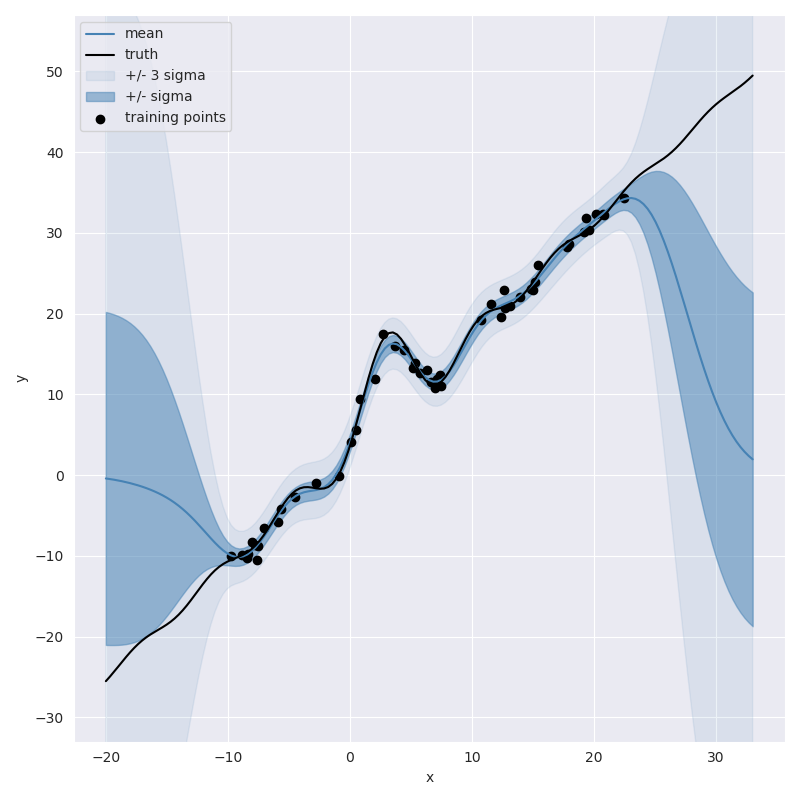From this plot we can see that the resulting model does a pretty good job of capturing the non-linearity of the sinc function in the vicinity of training data, including reasonable looking uncertainty estimates. However, you wouldn’t want to use this model to extrapolate outside of the training domain since the predictions quickly return to the prior prediction of 0.

To improve the model’s performance outside of the training domain we may want to introduce a systematic term to the model. For example instead of simply saying “the unknown function is non-linear but smooth” we may want to say “the unknown function is linear plus a non-linear smooth component.” We can do this by introducing a polynomial term into the covariance function.

More specifically we can define a covariance function which represents $$p(x) = a x + b$$ by first placing priors on the values $$a$$ and $$b$$,

\begin{align}\begin{aligned}a \sim \mathcal{N}(0, \sigma_a^2)\\b \sim \mathcal{N}(0, \sigma_b^2)\end{aligned}\end{align}

leading to the covariance function:

$\mbox{linear}(x, x') = \sigma_a^2 x x' + \sigma_b^2.$

Where the first term captures the covariance between $$x$$ and $$x'$$ from the linear component and second term captures the covariance from the common offset.

Now we can assemble this into a new covariance function,

$\mbox{cov}(x, x') = \mbox{linear}(x, x') + \mbox{sqr_exp}(|x - x'|) + \mbox{meas_noise}(x, x'),$

create a Gaussian process from it and plot the resulting predictions which look like: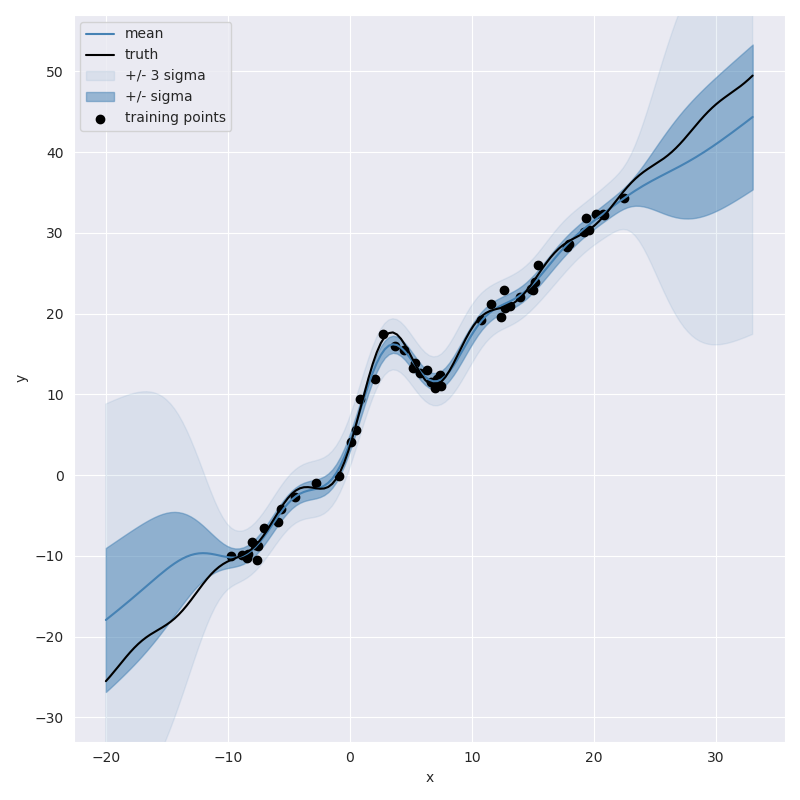This plot shows that the model’s ability to extrapolate has been been significantly improved.

##### Implementation in albatross¶

One of the primary goals of albatross is to make iterating on model formulations like we did in the examples above easy and flexible. All the components mentioned earlier are already pre-defined. The creation of basic Gaussian process which consists of a squared exponential term plus measurement noise, for example, can be constructed as follows,

IndependentNoise<double> independent_noise;
SquaredExponential<EuclideanDistance> squared_exponential;
auto model = gp_from_covariance(sqrexp + independent_noise);


Similarly we can build a model which includes the linear (polynomial) term using,

IndependentNoise<double> independent_noise;
SquaredExponential<EuclideanDistance> squared_exponential;
Polynomial<1> linear;
auto model = gp_from_covariance(linear + sqrexp + independent_noise);


We can inspect the model and its parameters,

std::cout << covariance.pretty_string() << std::endl;


Which shows us,

((polynomal_1+independent_noise)+squared_exponential[euclidean_distance])
{
{"sigma_independent_noise", 1},
{"sigma_polynomial_0", 100},
{"sigma_polynomial_1", 100},
{"sigma_squared_exponential", 5.7},
{"squared_exponential_length_scale", 3.5},
};


then condition the model on random observations, which we stored in data,

model.fit(data);


and make some gridded predictions,

const int k = 161;
const auto grid_xs = uniform_points_on_line(k, low - 2., high + 2.);
const auto predictions = model.predict(grid_xs);


Here are the resulting predictions when we have only two noisy observations,

##### 2 Observations¶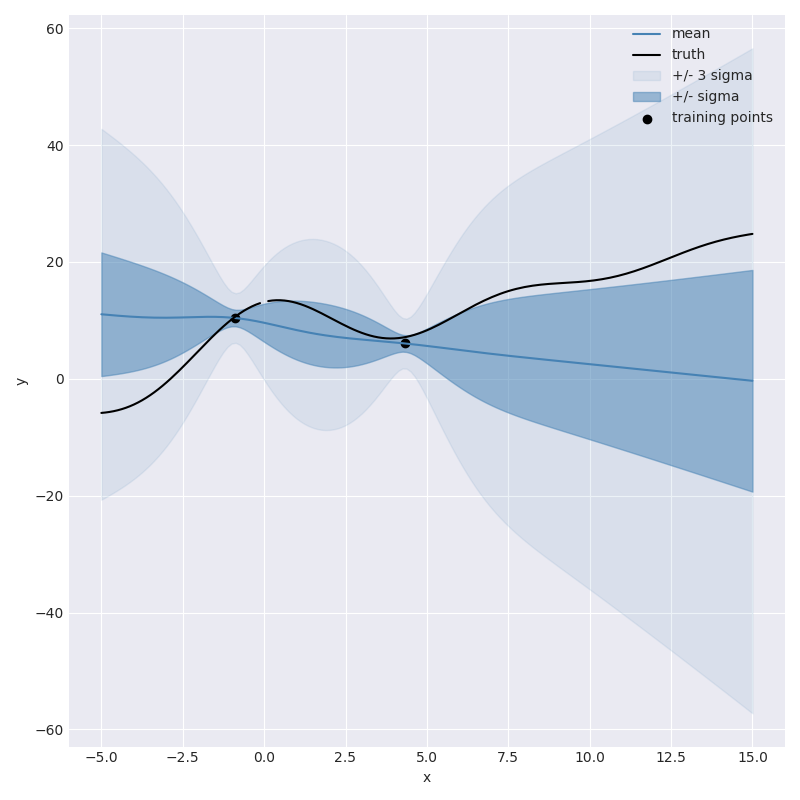not great, but at least it knows it isn’t great. As we start to add more observations we can watch the model slowly get more confident,

##### 5 Observations¶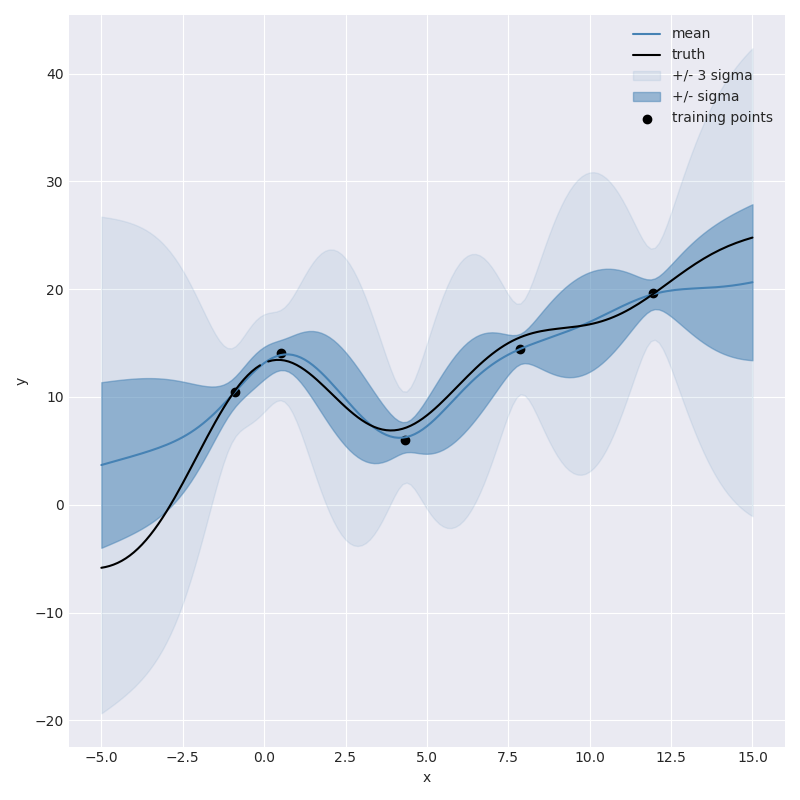##### 10 Observations¶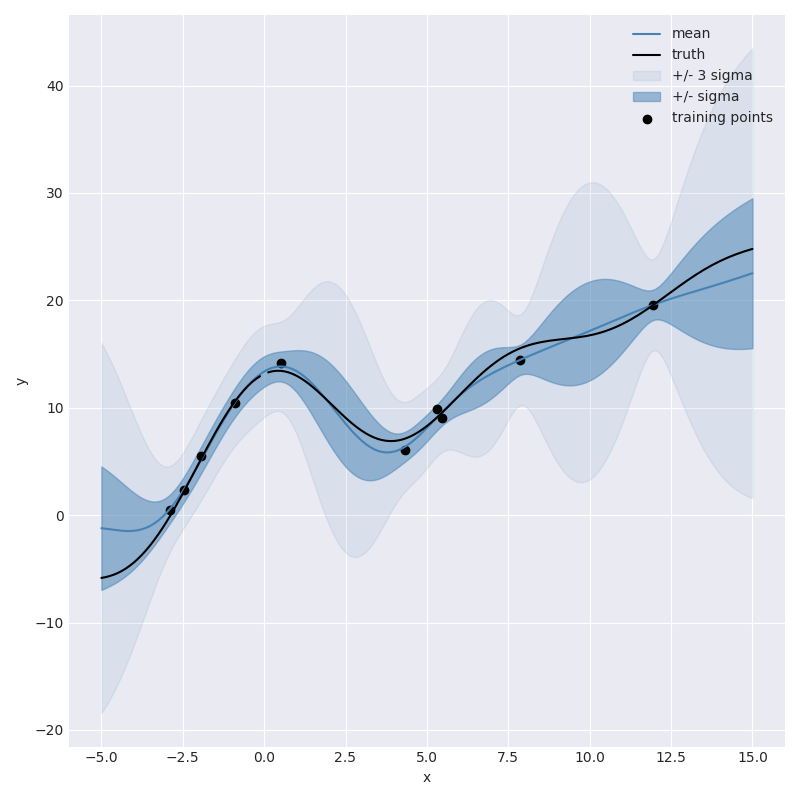##### 30 Observations¶#### Temperature Example¶

##### Introduction¶

Gaussian processes are quite popular in geostatistics where models such as Kriging are used to interpolate spatial fields. The idea is very similar to the 1D Example, there is some unknown function (in our example it will be temperature as a function of location) and you have noisy observations of the function (from weather stations) which you would like to use to make estimates at new locations.

##### Temperature Data¶

For this example we’ll use the Global Summary of the Day (GSOD) data to produce an estimate of the average temperature for a given day over the continental United States (CONUS).

Here are the GSOD observation of average temperature for May 1st, 2018,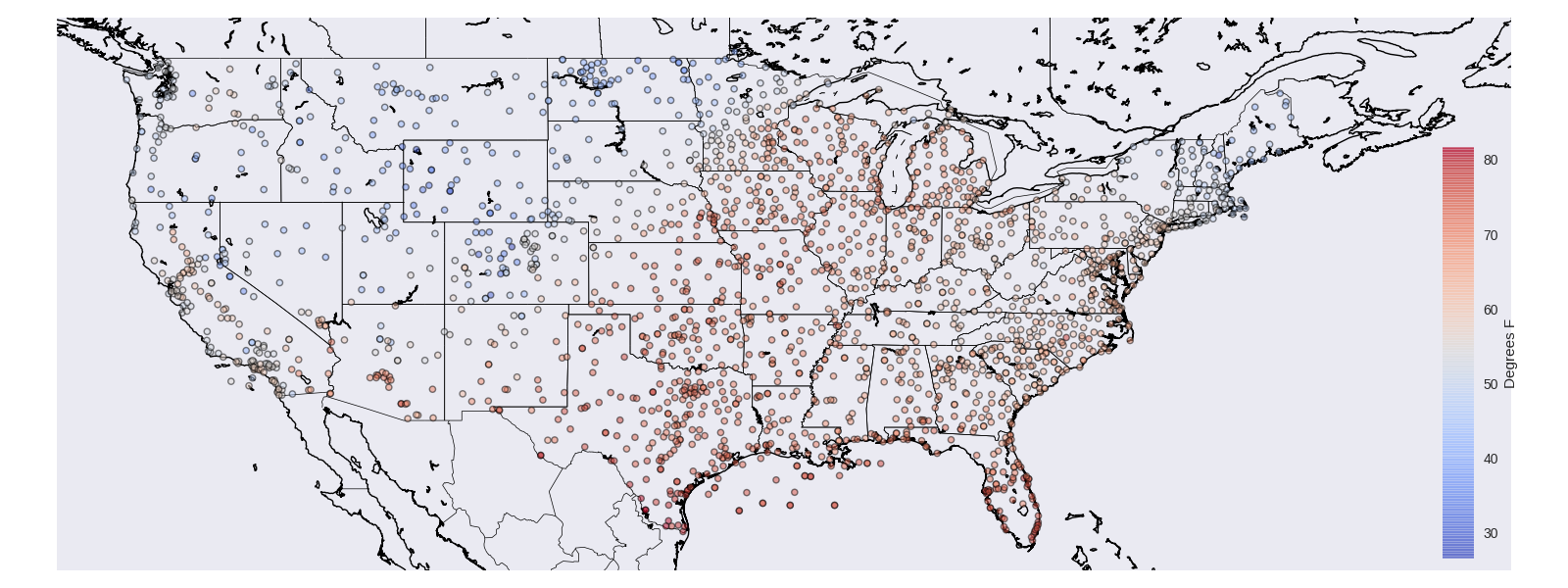##### Temperature Model¶

We’re going to build our temperature model to incorporate the following priors about how temperature should be have.

• Temperature at neighboring locations should be similar.
• There is some non-zero average temperature.
• Temperature decreases with elevation

To accomplish this we can build a covariance function that is not dissimilar from the one used in the 1D Example. We can start with measurement noise,

IndependentNoise<Station> noise(2.0);


Which states that each weather station makes observations of the average temperature that includes noise with a standard deviation of $$2$$ degrees.

We can then define a mean value for our field. In reality we might expect that the mean average temperature for a day would vary geographically, for this model we’ll simply claim that there is some mean average temperature for all of CONUS,

Constant mean;


Now we capture the spatial variation by saying that locations near each other in terms of great circle distance will be similar,

// The angular distance is equivalent to the great circle distance
SquaredExponential<StationDistance<AngularDistance>> angular_sqrexp;


Then add that stations at different elevations will be dissimilar,

// Radial distance is the difference in lengths of the X, Y, Z
// vectors, which translates into a difference in height so
// this term means "station at different elevations will be less correlated"
Exponential<StationDistance<RadialDistance>> radial_exp;


These can be combined to get our final covariance function,

auto spatial_cov = angular_sqrexp * radial_exp;
auto covariance = mean + noise + spatial_cov;


For the full implementation details see the example code.

##### Elevation Scaling¶

Then as we’d mentioned we’d like to include the concept of temperature decreasing with elevation. To do this we can create a scaling term which scales the mean value based on the elevation.

$\mbox{elevation_scaling}(h) = 1. + \alpha \left(H - h\right)_{+}.$

Where $$\left(\cdot\right)_{+}$$ could also be written $$\mbox{max}(0, \cdot)$$ and returns the argument if positive $$0$$ otherwise. The resulting function will decrease at a rate of $$\alpha$$ until $$h >= H$$ afterwhich the scaling term will flatten out to a constant value of $$1$$. By multiplying this term through with the mean we get a prior which will allow for higher temperatures at low elevations.

Here is how you can implement such a scaling function in albatross.

class ElevationScalingFunction : public albatross::ScalingFunction {
public:

ALBATROSS_DECLARE_PARAMS(elevation_scaling_center, elevation_scaling_factor);

ElevationScalingFunction(double center = 1000., double factor = 3.5 / 300) {
elevation_scaling_center = {center, UniformPrior(0., 5000.)};
elevation_scaling_factor = {factor, PositivePrior()};
};

std::string get_name() const { return "elevation_scaled"; }

double _call_impl(const Station &x) const {
// This is the negative orientation rectifier function which
// allows lower elevations to have a higher variance.
const double center = elevation_scaling_center.values;
const double factor = elevation_scaling_factor.value;
return 1. + factor * fmax(0., (center - x.height));
}
};

// Scale the constant temperature value in a way that defaults
// to colder values for higher elevations.
ScalingTerm<ElevationScalingFunction> elevation_scalar;
auto elevation_scaled_mean = elevation_scalar * mean;
auto covariance = elevation_scaled_mean + noise + spatial_cov;

##### Gridded Predictions¶

Now that we’ve defined the covariance function we can let albatross do the rest!

auto model = gp_from_covariance(covariance);
model.fit(data);
const auto predictions = model.predict(grid_locations);


Here we created a Gaussian process from the covariance function, fit the model using the GSOD data and then made predictions on a grid. The predictions hold information about the mean and variance of the resulting estimates. We can look at the mean of the estimates,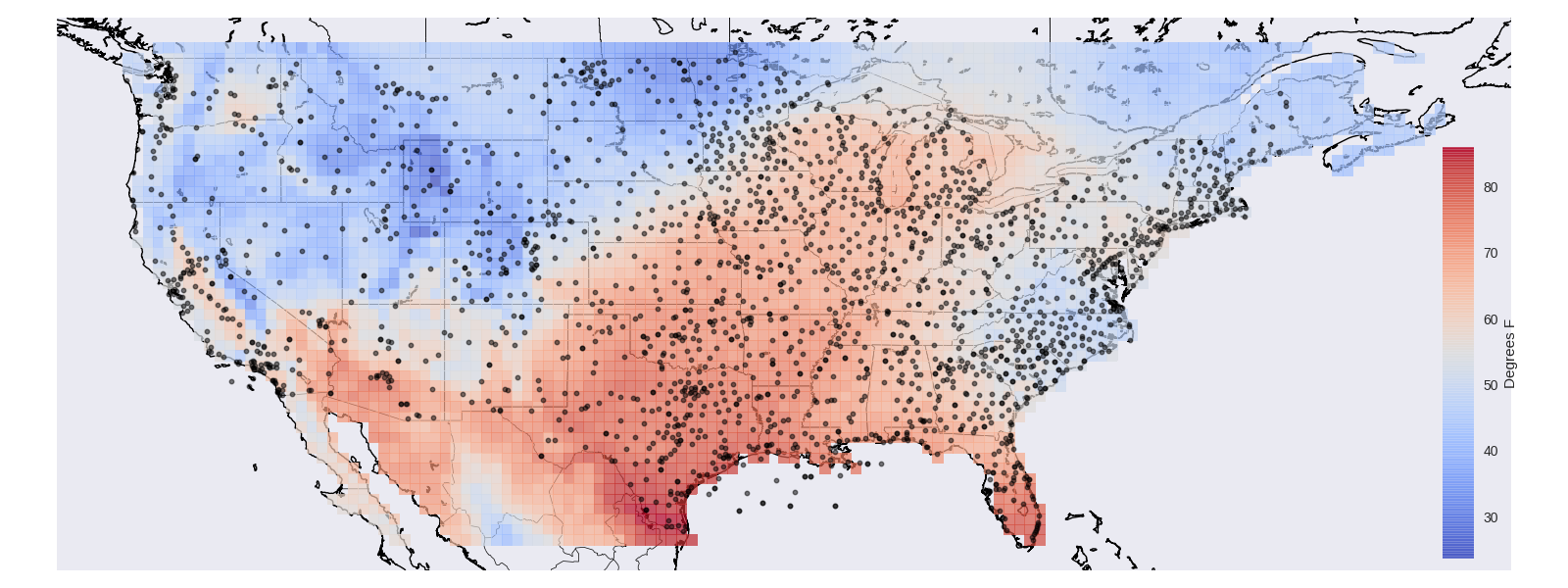and perhaps more interestingly we can also get out the variance, or how confident the model is about its predictions,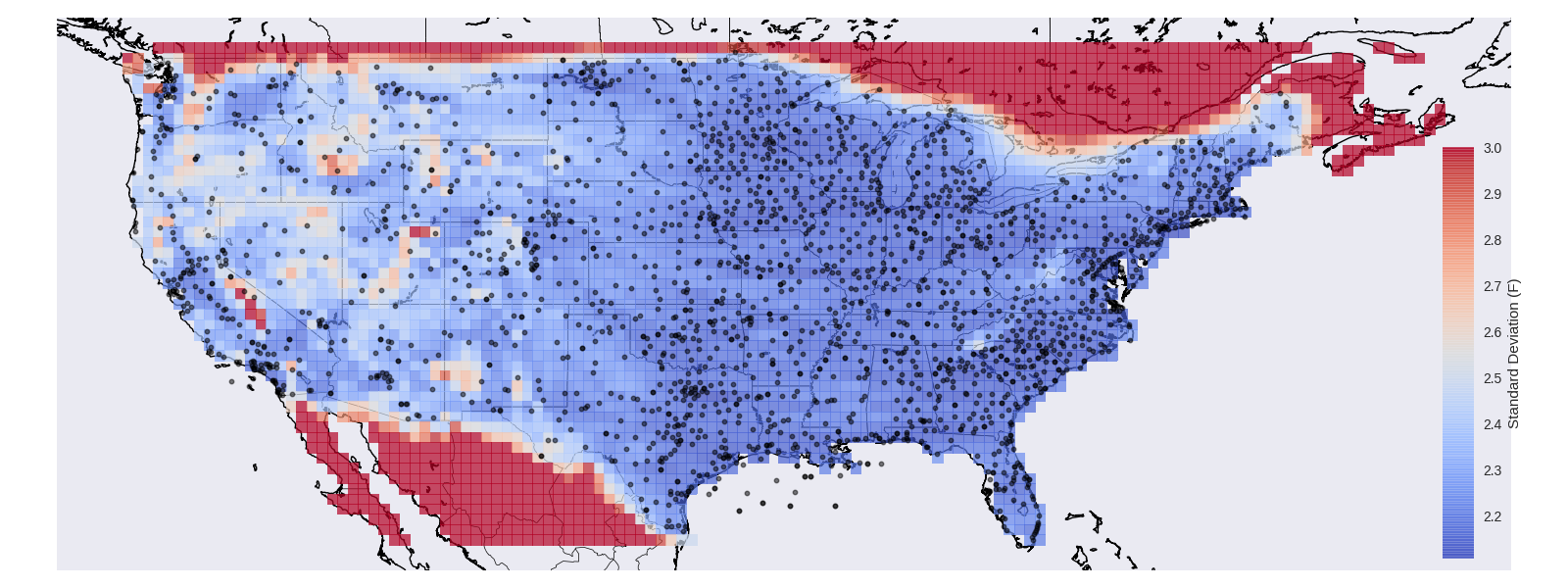Notice that the model is capable of realizing that it’s estimates should be trusted less in mountainous regions!

If you want to run this on your own you can build the temperature_example target:

make temperature_example && ./examples/temperature_example -input ../examples/temperature_example/gsod.csv  -predict ../examples/temperature_example/prediction_locations.csv -thin 10 -output ./temperature_predictions.csv
python ../examples/temperature_example/plot_temperature_example.py ../examples/temperature_example/gsod.csv ./temperature_predictions.csv


#### Datasets¶

##### Regression Datasets¶

One of the core data types in albatross is the RegressionDataset. These objects are built to store a set of measurements which we use all over albatross. There are a number of additional helper methods in the RegressionDataset, but you can think of it as a simple struct:

template <typename FeatureType>
struct RegressionDataset {
std::vector<FeatureType> features;
MarginalDistribution targets;
}


Here we see that there is a vector of features. Each feature is meant to be an object which fully describes a measurement and each measurement consists of the actual value and an optional variance. These measurements are held in a MarginalDistribution which looks something like this:

struct MarginalDistribution {
Eigen::VectorXd mean;
Eigen::DiagonalMatrix<double, Eigen::Dynamic> variance;
}


The temperature dataset is a good example of how data is stored. That dataset consists of a large number of average daily temperature measurements at stations spread across the continental US. In this case we need to know things about where each measurement was taken which we store in a Station struct,

struct Station {
double lat;
double lon;
double height;
}


The full dataset is then a RegressionDataset<Station> and we could do things like print the i th temperature measurement and where it was observed:

const RegressionDataset<Station> temperature_dataset = make_dataset();
std::cout << temperature_dataset.features[i].lat << " ";
std::cout << temperature_dataset.features[i].lon << " ";
std::cout << temperature_dataset.targets.mean[i] << std::endl;


There are some useful utility functions for manipulating these datasets. We can subset the dataset,

std::vector<std::size_t> first_few_odd_indices = {1, 3, 5, 7};
const RegressionDataset<Station> first_few_odd =
subset(temperature_dataset, first_few_odd_indices);


Or concatenate two datasets:

RegressionDataset<Station> ca = make_california_dataset();
RegressionDataset<Station> ny = make_new_york_dataset();
RegressionDataset<Station> both = concatenate_datasets(ca, ny);


You can actually concatenate datasets of different types as well, which will result in a new dataset which uses mapbox variant to store the combined types.

RegressionDataset<Station> ca = make_california_dataset();
RegressionDataset<int> constraints = make_constraint_dataset();
RegressionDataset<variant<Station, int>> both = concatenate_datasets(ca, constraints);


Finally, if you’ve written serialization routines for the types involved (see cereal and ) you can then dump your dataset to csv:

std::ofstream ofs("example.csv");
write_to_csv(ofs, temperature_dataset);


#### Group By : Split Apply Combine¶

The split apply combine workflow is something that is heavily used in the popular python package pandas and which we’ve borrowed in albatross. The general idea is that many data manipulation operations can be broken into three steps in which a dataset is first split apart into groups, a function is then applied to each of the groups and the result is recombined into a new dataset.

This technique can be used in albatross using syntax that ends up very similar to that of pandas

dataset.group_by(my_criteria).apply(some_operation).combine();

##### Group By¶

The grouping (and applying) can be done using anything callable which takes a single FeatureType as an argument, for example:

int my_criteria(const double &x) { return round(x); }

struct MyCritera {
int operator() (const double &x) {
return my_criteria(x);
}
}

void examples() {
RegressionDataset<double> dataset = make_dataset();

// using a free function
dataset.group_by(my_criteria);
// using a lambda
dataset.group_by([](const auto &x){return my_criteria(x);});
// using a callable object
MyCritera criteria;
dataset.group_by(criteria);
}


The result of group_by(f).groups() can be treated as if it were a std::map, for example to get the third group:

auto dataset_3 = dataset.group_by(my_criteria).groups();


Not all operations can be efficiently done working with the grouped datasets, for these cases you may find it helpful to work directly with the indices of each group:

std::vector<std::size_t> inds_3 = dataset.group_by(my_criteria).indexers();


The group_by technique can also be used directly on vectors:

std::vector<double> values = make_values();
group_by(values, my_criteria);


In this case the groups() will return a map from int to std::vector<double>.

##### Apply¶

Similar to group_by an apply function can be anything callable and should take either the key value pair or just the value as arguments and can (optionally) return a new object. In other words an apply function should have one of the following signatures.

ApplyType f(KeyType &key, ValueType &value)
The result will be a new map-like from KeyType to ApplyType
auto sum = [](const int &key, const RegressionDataset<double>& value)
{ return value.targets.mean.sum(); };
std::map<int, double> sums = dataset.group_by(my_criteria).apply(sum);

void f(KeyType &key, ValueType &value)
The return type will be void.
auto print_sum = [](const int &key, const RegressionDataset<double>& value)
{ std::cout << key << " : " << value.targets.mean.sum() << std::endl; };
dataset.group_by(my_criteria).apply(print_sum);

ApplyType f(ValueType &value)
The result will be a new map-like from KeyType to ApplyType
auto sum = [](const RegressionDataset<double>& value)
{ return value.targets.mean.sum(); };
std::map<int, double> sums = dataset.group_by(my_criteria).apply(sum);

void f(KeyType &key, ValueType &value)
The return type will be void.
auto print_sum = [](const RegressionDataset<double>& value)
{ std::cout << value.targets.mean.sum() << std::endl; };
dataset.group_by(my_criteria).apply(print_sum);


For example, we could do something like:

RegressionDataset<Bar> dataset;
auto get_foo = [](const Bar &bar) { return Foo(bar); };
dataset.group_by(get_foo).apply(f);


In this situation the ValueType = RegressionDataset<Bar> and KeyType = Foo.

auto can be used for the argument types in which case a single argument is assumed to be a ValueType. For example,

dataset.group_by(get_foo).apply([](const auto &data) {return f(data);});

##### Combine¶

In the apply step there are very few restrictions on what can be returned from an apply function. When it comes to the combine step however, there are a few restrictions. Namely combine only supports RegressionDataset<>, std::vector<> and double types.

In this example you can see how you could start with a dataset, split it into groups compute some metric for each group and recombine into a vector of the results:

auto compute_something = [](const RegressionDataset<Bar> &data) -> double {
double something = data.features.foo;
return something;
}

Eigen::VectorXd results = dataset.group_by(get_group).apply(compute_something).combine();

##### Motivational Example¶

One common pattern when working with data is the need to break a dataset apart and do something with each of the resulting groups. For example, in the group_by_example we built a dataset which contains a bunch of people defined by their age and gender:

struct Person {
enum Gender {FEMALE, MALE};

Gender gender;
int age;
};


In albatross we store data using the RegressionDataset<> type which consists of a vector of features and an Eigen::VectorXd of targets. You can think of the features as an object containing all the information you need to describe some measurement and the targets as containing the actual measurements.

We might then, for example, want to take our dataset of people and print out the average salary depending on the gender. Here’s how you might do that manually:

std::size_t female_count = 0;
double female_average = 0.;
std::size_t male_count = 0;
double male_average = 0.;

for (std::size_t i = 0; i < dataset.size(); ++i) {
if (dataset.features[i].gender == Person::Female) {
female_average += dataset.targets.mean[i];
++female_count;
} else {
male_average += dataset.targets.mean[i];
++male_count;
}
}

female_average /= female_count;
male_average /= male_count;

std::cout << "female : " << female_average << std::endl;
std::cout << "male : " << male_average << std::endl;


There are several issues with this though. If there are no males (or females) in the dataset we’ll end up dividing by zero. Also, if you are dealing with more than two options the details of the for loop (which are already a bit difficult to follow) could get very complicated. Instead we can use the group_by and apply methods to come up with an alternative approach:

const RegressionDataset<Person> dataset = make_data();

auto get_gender = [](const auto &f){return f.gender;};

auto print_average_salary = [](const auto &gender, const auto &dataset) {
std::cout << to_string(gender) << "  :  " << dataset.targets.mean.mean() << std::endl;
};

dataset.group_by(get_gender).apply(print_average_salary);


Not only will this avoid the pitfall of missing groups, but the split-apply approach forces the use of smaller helper functions which ends up making everything much easier to read.

#### Gaussian Processess¶

Here we describe how to build and work with Gaussian processes in albatross, this assumes a basic understanding of what Gaussian processes are. The python package scikit learn has a good practical introduction and for a complete theoretical explanation the book Gaussian Process Regression is an excellent resource. It could also be worth going through the 1d example and the temperature example to get a general idea of how Gaussian processes can be applied. In this section we’ll focus on how to build a Gaussian process in albatross, in particular how to create covariance functions, build a GP from them and how to use the model to make predictions.

##### Basic Workflow¶

TLDR; Here is an example work flow in which we create a GP, tune its parameters to maximize the leave one out cross validated likelihood, then fit the model and use it to make predictions of unobserved data.

RegressionDataset<double> training_data = make_training_data();
RegressionDataset<double> evaluation_data = make_evaluation_data();

const IndependentNoise<double> independent_noise;
const SquaredExponential<EuclideanDistance> squared_exponential;
const auto covariance = squared_exponential + independent_noise;
auto gp = gp_from_covariance(covariance);

albatross::LeaveOneOutLikelihood<> loo_nll;
const auto tuned_params = get_tuner(gp, loo_nll, training_data).tune();
gp.set_params(tuned_params);

const auto fit_model = gp.fit(training_data);
const Eigen::VectorXd prediction = fit_model.predict(evaluation_data.features).mean();

const double rmse = root_mean_square_error(prediction, evaluation_data.targets);

std::cout << "Evaluation RMSE: " << rmse << std::endl;

##### Covariance Functions¶

A Gaussian process (GP) is defined by its covariance function (and occasionally by a mean function). The covariance function is responsible for describing the relationship between any two variables and is used to build a full description of the training data and subsequently describe how the test data depends on the training data which can be used to make predictions at un-observed locations.

To start, here is how one would build a very basic but functional covariance function which works with one dimensional data,

IndependentNoise<double> independent_noise;
SquaredExponential<EuclideanDistance> squared_exponential;
const auto covariance = squared_exponential + independent_noise;


The resulting variable covariance will be be derived from the albatross::CovarianceFunction<> type which contains a large number of helper methods. In particular you can treat the resulting type as callable object which will return the covariance between any two data points:

double x = 0.;
double y = 1.;
double cov = covariance(x, y);


Similarly you can compute the covariance matrix between all points in a vector,

std::vector<double> points = {0., 1., 2.};
// Creates a 2 x 2 matrix
Eigen::MatrixXd cov = covariance(points);

std::vector<double> other_points = {4., 5.};
// Creates a 3 x 2 matrix
Eigen::MatrixXd cross_cov = covariance(points, other_points);


Covariance functions often depend on parameters. In this case we have the independent_noise function which will have a parameter representing the magnitude of the measurement noise. We can inspect it using get_params():

std::cout << pretty_params(independent_noise.get_params()) << std::endl;

{
{"sigma_independent_noise", 1},
};


Notice that the sum of independent_noise and squared_exponential will consist of the concatenation of both their params,

std::cout << pretty_params(covariance.get_params()) << std::endl;


Which would result in,

{
{"sigma_independent_noise", 1},
{"sigma_squared_exponential", 5.7},
{"squared_exponential_length_scale", 3.5},
};

###### Operators¶

We already saw how you can sum covariance functions together to get a new function, but you can also take the product,

auto sum = foo + bar;
auto prod = foo * bar;


One situation where you may want to use the product of two covariance functions is when you want to decorrelate what would otherwise be correlated terms. For example, when dealing with spatial and temporal data (such as the temperature example) you may want a term (spatial) which says “Nearby locations will have a similar temperature” and another term (temporal) which says “Temperature changes over the course of time”. Which could be combined into another covariance function (spatio_temporal = spatial * temporal) which says, “Measurements taken at similar locations and times will be similar.”

###### Writing Your Own¶

The covariance functions in albatross use the Curiously Recurring Template Pattern (CRTP) which makes defining them slightly different from the standard inheritence pattern in C++. For example, to write your own simple covariance function you could start with a definition such as,

class Simple : public CovarianceFunction<Simple> {
public:
double _call_impl(const X &x, const X &other) const {
return 1.;
}
}


The resulting covariance function will be callable with any arguments that are of type X but will otherwise result in a compile time failure:

Simple simple;
X x;
Y y;
// this is fine:
double xx = simple(x, x);
// this would fail to compile:
double xy = simple(x, y);


Notice that by defining a _call_impl method in your covariance function the base class enabled the corresponding call operator(s). This is the primary reason for the use of CRTP, namely the CovarianceFunction<Derived> base class is capable of inspecting the Derived class and enabling methods such as the operator() depending on which _call_impl methods have been defined. This next example is not actually valid C++, but it might help to think of the CovarianceFunction class as an abstract class with signature.

class CovarianceFunction {
public:
template <typename X, typename Y>
virtual double _call_impl(const X &, const Y &) const = 0

template <typename X, typename Y>
double operator()(const X &x, const Y &y) const {
return this->_call_impl(x, y);
}
}


Covariance functions can be parametrized, there are several ways to accomplish this but the ALBATROSS_DECLARE_PARAMS is likely your best bet:

class Simple : public CovarianceFunction<Simple> {

ALBATROSS_DECLARE_PARAMS(simple_sigma);

Simple(const double &sigma) {
simple_sigma = {sigma, PositivePrior()};
}

public:
double _call_impl(const X &x, const X &other) const {
return simple_sigma.value * simple_sigma.value;
}
}


any parameters you define will then be gettable and settable using the get_params() and set_params() methods (as well as a number of other helper methods) in both the covariance function itself and any compositions including it as well as any Gaussian processes which use it. Also worth noting that there are a number of other priors you can choose from.

If you are writing your own covariance functions you might find it helpful to take a look at some of the examples and the predefined covariance functions.

CRTP definitely adds to the complexity but it enables some of the most powerful features in albatross; the ability for covariance functions to work with arbitrary custom types and the composition of covariance functions through + and * operators.

###### Multiple Types¶

Covariance functions are not restricted to work with a single type, in fact this is one of the more powerful features in albatross. For example you could write a CovarianceFunction like this:

class Both : public CovarianceFunction<Both> {

double _call_impl(const X &x, const X &other) const {
return 3.;
}

double _call_impl(const X &x, const Y &y) const {
return 5.;
}

double _call_impl(const Y &y, const Y &other) const {
return 7.;
}

}


Which we can then sum together with Simple and the behavior changes,

Simple simple;
Both both;
auto sum = simple + both;

sum(x, x) // 4.
sum(x, y) // 5.
sum(y, y) // 7.


Once you’ve defined a covariance function you can also call it with a variant,

variant<X, Y> vx = x;
variant<X, Y> vy = y;

sum(x, x) == sum(vx, vx);
sum(x, y) == sum(vx, vy);
sum(y, y) == sum(vy, vy);

##### Auto Is Your Friend¶

One of the drawbacks to CRTP is that the resulting types can be extremely verbose. Take the example above and note the use of auto sum = simple + both. The actual type of sum in this case would be SumOfCovarianceFunctions<Simple, Both>. Not too bad, but you can see how if you begin building covariance functions with multiple terms you quickly end up with very complicated types. Thankfully the use of auto should keep you from ever needing to actually know the underlying type.

#### Custom Models¶

##### Defining a Model¶

The ModelBase class in albatross uses the Curiously Recurring Template Pattern (CRTP) which makes defining them slightly different from the standard inheritence pattern in C++.

In general an albatross model requires defining _fit_impl, _predict_impl and a struct Fit<MyModel> which is in charge of storing any coefficients.

##### Fit Method¶

To get model.fit(dataset) to work you need to add a _fit_impl method to your class, this fit implementation needs to take a vector of features and corresponding targets (often measurements) and needs to return a Fit<ModelType> object holding any information required to make predictions.

class ModelType : public ModelBase<ModelType> {

Fit<ModelType> _fit_impl(const std::vector<FeatureType> &features,
const MarginalDistribution &targets) const;

}


The FeatureType here can be which ever type your problem requires and you can have multiple _fit_impl methods for different types in the same model. Templated _fit_impl methods also work.

##### Predict Method¶

To get model.predict(features) to work you need to add a _predict_impl method to your class, this predict implementation needs to take a vector of features and a Fit<ModelType> and needs to return either an Eigen::VectorXd (mean only), MarginalDistribution (mean and variance) or JointDistribution (mean and covariance).

class ModelType : public ModelBase<ModelType> {

JointDistribution _predict_impl(const std::vector<FeatureType> &features,
const Fit<ModelType> &fit,
PredictTypeIdentity<JointDistribution>) const;

}


In this case above we’ve implemented predict to return a JointDistribution which holds the mean prediction as well as a full covariance. A JointDistribution can be converted into a MarginalDistribution by taking the diagonal of the covariance matrix and a MarginalDistribution can be converted into a mean only prediction (Eigen::VectorXd) by simply taking the mean of the distribution. As a result, by implementing predict for a JointDistribution you will be able to call all of the following.

const auto prediction model.fit(dataset).predict(features);
JointDistribution joint_pred =prediction.joint();
MarginalDistribution marginal_pred = prediction.marginal();
Eigen::VectorXd mean_pred = prediction.mean();


If you define _predict_impl with a MarginalDistribution instead, then you’ll find that you can call,

MarginalDistribution marginal_pred = prediction.marginal();
Eigen::VectorXd mean_pred = prediction.mean();


but calling prediction.joint(); would result in a compile time error. Similarly if you just define the mean only version then asking for anything other than prediction.mean() will result in a compile time error.

We saw above that you could implement the JointDistribution version and have access to all the predict types, but that is often inefficient. Instead you may want to impelement specialized version for each of the predict types. This is what is done in for the Gaussian processes (see gp.hpp). The desire to have specialized predict types is what led to the mysterious PredictTypeIdentity<> argument, which is required to allow overridable _predict_impl methods with different return types.

##### Fit Type¶

The fit type needs to be a specialization of the Fit<> struct. The idea is that by forcing the output of _fit_impl to be a custom type we can subsequently make model types constant, which gives us peace of mind that there isn’t accidentally some state that get’s stored in a model which would cause two calls to fit to produce different results.

Once you’ve defined the Fit<> you shouldn’t ever need to actually inspect that type, that should be left to the internals of albatross. Instead you are encouraged to use auto,

const auto fit_model = model.fit(dataset);


or write everything as one liners.

const Eigen::VectorXd mean = model.fit(dataset).predict(features).mean();


Here’s an illustration of the actual types that would result from a typical model workflow:

const ModelType model = make_my_model();
const FitModel<ModelType, Fit<ModelType>> fit_model = model.fit(dataset);
const Prediction<ModelType, FeatureType, Fit<ModelType>> prediction = fit_model.predict(features);
const JointDistribution joint_prediction = prediction.joint();


Again, thanks to auto type declarations you shouldn’t need to actually know these types but it may be helpful to get a glimpse of what’s happening under the hood. This chain of types is what allows albatross to keep track of how exactly you’re using a model and decide (at compile time) the most efficient methods to use.

##### Example¶

Here’s an example of a model which always returns the mean of the training data.

struct Fit<MeanModel> {
double mean;
}

class MeanModel : public albatross::ModelBase<MeanModel> {
public:

using FitType = Fit<MeanModel>;

std::string get_name() const { return "mean"; }

template <typename FeatureType>
FitType _fit_impl(const std::vector<FeatureType> &features,
const MarginalDistribution &targets) const {
FitType model_fit = {targets.mean.mean()};
return model_fit;
}

template <typename FeatureType>
Eigen::VectorXd _predict_impl(const std::vector<FeatureType> &features,
const FitType &fit,
PredictTypeIdentity<Eigen::VectorXd>) const {
Eigen::VectorXd output(features.size());
output.fill(fit.mean);
return output;
}
}


While defining your own model isn’t as simple as standard inheritence , the benefits are large. Once you’ve defined a model using the ModelBase class you can immediately start using all the tools built around it, things such as cross validation, outlier detection using RANSAC, and tuning tuning.

#### Crossvalidation¶

If you’re using this package it’s probably safe to say you’re interested in building a statitical model and plan on training with some data and making predictions for another. During the process of you’ll invariable end up wondering how well the model work. It could be that you have multiple models and wnat to know which model will work best. Or could simply be that you’re curious how well your model will work in real life.

This is where cross validation steps in. The motivation is relatively simple: Fitting your model and testing it on the same data is cheating. Cross validation is an approach for getting a more representative estimate of a model’s generalization error, or the ability of your model to predict things it hasn’t seen. The process consists of spliting your data into groups, holding one group out, fitting a model to the remaining data and predicting the held out group, then repeating for all groups. The result will be out of sample predictions for all the data in your dataset which can be compared to the truth and used with your favorite metrics

##### Example Usage¶
// Get the cross validated marginal predictions in the original order;
model.cross_validate().predict(dataset, get_group).marginal();
// Get the cross validated marginal predictions by group;
model.cross_validate().predict(dataset, get_group).marginals();


#### Tuning¶

So you’ve built a model, but somewhere in the process it required adding some nuisance parameters. Sometimes these parameters have realtively well known values, but often that’s not the case. In such situations it’s common to tune (or optimize) the parameters in your model.

Here is an example of how you could tune your model’s parameters to maximize the leave one out cross validated likelihood,

LeaveOneOutLikelihood<MarginalDistribution> loo_nll;
const auto tuner = get_tuner(model, loo_nll, data);
auto tuned_params = tuner.tune();
model.set_params(params);


Tuning in albatross piggy backs off of nlopt. There are a lot of ways to customize the tuning process most of which can be accomplished by accessing the tuner.optimizer member.

##### Priors¶

Model parameters often have priors, you may know for example that one of them is always between 0 and 1. Others may be strictly positive. This sort of information can all get stored in an albatross model. Here, for example, is how you might define your model to expose the parameters,

class MyModel : public ModelBase<MyModel> {
MyModel() {
params_["foo"] = {0.5, UniformPrior(0., 1.)};
params_["bar"] = {10, PositivePrior()};
}
}


If you’ve properly defined the parameters in your model (and picked a compatible nlopt algorithm) tuning will respect the parameter bounds. Metrics such as the LeaveOneOutLikelihood will also include the likelihood of the parameters.

#### Markov Chain Monte Carlo¶

Albatross has an implementation of an ensemble Bayesian sampler based off the paper Ensemble samplers with affine invariance which was inspired by the emcee package in python.

The idea is that by defining a function which computes the likelihood (or something proportional to the likelihood) you can then produce samples from the distribution of parameters. To do so the algorithm iteratively proposes new parameters and accepts the new proposed parameters based off the ratio of the current parameters likelihood with the proposed parameters likelihood.

Here is how you could run the ensemble sampler for some arbitrary likelihood function,

auto proportional_to_ll(const std::vector<double> &params) {
// compute and return a value proportional to the likelihood of the params
}

std::default_random_engine gen(0);
std::vector<std::vector<double>> initial_params = get_initial_params();
std::size_t max_iterations = 1000;
const auto ensemble_samples =
ensemble_sampler(proportional_to_ll, initial_params, max_iterations, gen);


There are some helper functions when dealing directly with albatross models,

std::default_random_engine gen(0);
std::size_t walters = 32;
std::size_t max_iterations = 1000;
auto csv_callback = get_csv_writing_callback(model, "path_to_params.csv");
const auto samples = ensemble_sampler(model, dataset, walkers, max_iterations,
gen, csv_callback);


In this case the initial parameters will be directly extracted fro mthe model, and as the sampler runs the output will get stored in a csv file which can then be inspected using examples/plot_sampler_output.csv.

#### Sparse Gaussian Process Implementation Details¶

##### Introduction¶

Here we describe an approximation technique for Gaussian processes proposed in:

 Sparse Gaussian Processes using Pseudo-inputs Edward Snelson, Zoubin Ghahramani http://www.gatsby.ucl.ac.uk/~snelson/SPGP_up.pdf

Though the code uses notation closer to that used in this (excellent) overview of similar methods:

 A Unifying View of Sparse Approximate Gaussian Process Regression Joaquin Quinonero-Candela, Carl Edward Rasmussen http://www.jmlr.org/papers/volume6/quinonero-candela05a/quinonero-candela05a.pdf

We’ve implemented the FITC and PITC methods which rely on an assumption that all observations (or groups of observations) are independent conditional on a set of inducing points (aka common state) which allows us to factorize the training covariance matrix into a low rank term and a diagonal term which leads to significant reduction in computational complexity compared to a full Gaussian process.

The method starts with the standard Gaussian process prior over the observations,

$[\mathbf{f}] \sim \mathcal{N}(0, K_{ff})$

Where $$K_{ff}$$ is the covariance function evaluated at all the training locations. It then uses a set of inducing points, $$u$$, and makes some assumptions about the conditional distribution:,

$[\mathbf{f}|u] \sim \mathcal{N}\left(K_{fu} K_{uu}^{-1} u, K_{ff} - Q_{ff}\right)$

Where $$Q_{ff} = K_{fu} K_{uu}^{-1} K_{uf}$$ represents the variance in $$f$$ that is explained by $$u$$.

For FITC (Fully Independent Training Conditional) the assumption is that $$K_{ff} - Q_{ff}$$ is diagonal, for PITC (Partially Independent Training Conditional) that it is block diagonal. These assumptions lead to an efficient way of inferring the posterior distribution for some new location $$f^*$$,

$[f^*|f=y] \sim \mathcal{N}(K_{*u} \Sigma K_{uf} \Lambda^{-1} y, K_{**} - Q_{**} + K_{*u} \Sigma K_{u*})$

Where $$\Sigma = (K_{uu} + K_{uf} \Lambda^{-1} K_{fu})^{-1}$$ and $$\Lambda = {\bf diag}(K_{ff} - Q_{ff})$$ and $${\bf diag}$$ may mean diagonal or block diagonal. See Equation 24 in  for details. Regardless we end up with $$O(m^2n)$$ complexity instead of $$O(n^3)$$ of direct Gaussian processes.

##### QR Solver¶

The QR approach to the Sparse Gaussian process draws on the equivalence between the normal equations and QR solutions to the least squares problem and is outlined at length in:

 Stable and EfficientGaussian Process Calculations. Leslie Foster, Alex Waagen, Nabeela Aijaz, Michael Hurley, Apolonio Luis,Joel Rinsky, Chandrika Satyavolu, and Mailbolt Com. http://www.jmlr.org/papers/volume10/foster09a/foster09a.pdf

Here we’ll recap the approach they describe but with some minor changes to reflect specifics of our implementation.

Least squares consists of solving for $$x$$ which minimizes $$\lVert A x- b\rVert$$ with $$A \in \mathbb{R}^{n, m}$$, $$x \in \mathbb{R}^m$$, $$b \in \mathbb{R}^n$$ and $$n > m$$. There are several approaches that can be used to solve the problem. One approach uses the normal equations to acquire $$x_N = \left(A^T A\right)^{-1} A^T b$$. A theoretically equivalent, but more numerically stable, approach involves computing the QR decomposition $$A = QR$$, after which you can solve for $$x_{QR} = R^{-1} Q^T b$$.

##### Predictive Expectation¶

To see how this can be applied to prediction in the context of Sparse Gaussian processes we first look at the mean of prediction distribution,

$\begin{split}y^{*} &= Q_{*f}\left(\Lambda + Q_{ff}\right)^{-1}y \\ &= K_{*u} K_{uu}^{-1} K_{uf} \left(\Lambda + K_{fu} K_{uu}^{-1} K_{uf}\right)^{-1} y \\ &= K_{*u} \left(K_{uu} + K_{uf} \Lambda^{-1} K_{fu}\right)^{-1} K_{uf} \Lambda^{-1} y\end{split}$

We can break apart the inverted term into an inner product of an augmented tall skinny matrix,

$\begin{split}B &= \begin{bmatrix} \Lambda^{-1/2} K_{fu} \\ L_{uu}^T \end{bmatrix},\end{split}$

where $$K_{uu} = L_{uu} L_{uu}^T$$. Then we see that substituting $$B$$ back into the predicted mean gives,

$\begin{split}y^* &= K_{*u} \left(K_{uu} + K_{uf} \Lambda^{-1} K_{fu}\right)^{-1} K_{uf} \Lambda^{-1} y \\ &= K_{*u} \left(B^T B\right) B^T \Lambda^{-1/2} y \\ &= K_{*u} v\end{split}$

Where $$v = \left(B^T B\right) B^T \Lambda^{-1/2} y$$ is the information vector which is in the form of a normal equation solution to the system,

$\begin{split}\left\lVert B v - \begin{bmatrix}\Lambda^{-1/2} y \\ 0 \end{bmatrix} \right\rVert\end{split}$

We can then compute the QR decomposition of $$B$$ which, when using a column pivoting scheme gives $$B = QRP^T$$. In its full form the QR decomposition of $$B \in \mathbb{R}^{k, m}$$ consists of the orthonormal matrix $$Q \in \mathbb{R}^{k, m}$$, the upper triangular matrix $$R \in \mathbb{R}^{m, m}$$ and a permutation matrix $$P \in \mathbb{R}^{m, m}$$ which, by recognizing that the lower portion of the right hand side in our least squares problem is zero, can be broken into parts and used to solve for $$v$$,

$\begin{split}B &= \begin{bmatrix} Q_1 \\ Q_2 \end{bmatrix} R P^T \\ v &= P R^{-1} Q_1^T \Lambda^{-1/2} y\end{split}$
##### Predictive Covariance¶

Turning back to the predictive distribution, we see we now have

$\begin{split}[f^*|f=y] &\sim \mathcal{N}(K_{*u} v, K_{**} - Q_{**} + K_{*u} \Sigma K_{u*}) \\ &\sim \mathcal{N}(K_{*u} v, K_{**} - E_{**})\end{split}$

Where we use $$E_{**}$$ to represent the covariance which is explained via $$[u|y]$$. The two terms involved can be interpreted as the law of total variance or in other words, $$E_{**}$$ consists of the covariance that would be explained if you knew the inducing points perfectly, $$Q_{**} = K_{*u} K_{uu}^{-1} K_{u*}$$, minus the uncertainty of the inducing points, $$\Sigma$$, mapped to the predictions, $$K_{*u} \Sigma K_{u*}$$. Refactoring we have,

$\begin{split}E_{**} &= Q_{**} - K_{*u} \Sigma K_{u*} \\ &= K_{*u} K_{uu}^{-1} K_{u*} - K_{*u} \Sigma K_{u*} \\ &= K_{*u} L_{uu}^{-T} L_{uu}^{-1} K_{u*} - K_{*u} \left(B^T B\right)^{-1} K_{u*} \\ &= \left(L_{uu}^{-1} K_{u*}\right)^T \left(L_{uu}^{-1} K_{u*}\right) - K_{*u} \left(P R^T Q^T Q R P^T\right)^{-1} K_{u*} \\ &= \left(L_{uu}^{-1} K_{u*}\right)^T \left(L_{uu}^{-1} K_{u*}\right) - \left(R^{-T} P^T K_{u*}\right)^T \left(R^{-T} P^T K_{u*}\right) \\ &= V_{a}^T V_{a} - V_{b}^T V_{b}\end{split}$

this has the nice property of being composed entirely of inner products which will ensure that the resulting posterior covariance will always be symmetric.

##### Summary¶

Putting this all together we have the following algorithm for the QR approach to a Sparse Gaussian Process.

First we compute the square root of $$\Lambda$$. When $$\Lambda$$ is a pure diagonal matrix this is simple,

$\Lambda^{-1/2}_{ii} = 1 / \sqrt{\Lambda_{ii}}.$

When $$\Lambda$$ is a block diagonal matrix we use the LDLT decomposition of each block, $$\Lambda_{b} = P^T L D L^T P$$ which we can use to get,

$\Lambda_{b}^{-1/2} = D_b^{-1/2} L_b^{-1} P_b$

where $$D_b^{-1/2}$$ is the square root of a diagonal matrix and $$P_b$$ is the permutation matrix computed when performing the LDLT with pivoting.

Next we can compute the square root of $$K_{uu}$$ which above we represented by $$L_{uu}$$, but when implementing we’ll use the LDLT again which gives us,

$K_{uu}^{-1/2} = D_{uu}^{-1/2} L_{uu}^{-1} P_{uu}$

We can now form the matrix $$B$$ and compute its QR decomposition,

$\begin{split}B &= \begin{bmatrix} \Lambda^{-1/2} K_{fu} \\ L_{uu}^T \end{bmatrix} \\ &= \begin{bmatrix} Q_1 \\ Q_2 \end{bmatrix} R P^T\end{split}$

which we can use to get $$v = P R^{-1} Q_1^T \Lambda^{-1/2} y$$. At this point we can store, $$v$$, $$R$$, $$P$$ and $$K_{uu}^{-1/2}$$ to use for predictions.

A prediction can then be made by computing, $$V_a = K_{uu}^{-1/2} K_{u*}$$ and $$V_b = R^{-T} P^T K_{u*}$$ which we can then use to create,

$[f^*|f=y] \sim \mathcal{N}(K_{*u} v, K_{**} - V_a^T V_a + V_b^T V_b)$
##### Adding a Group¶

Our implementation of the Sparse Gaussian Process can be efficiently updated with new groups in an online fasion. In otherwords this allows you to do:

auto fit_model = model.fit(dataset_a);
fit_model.update_in_place(dataset_b);


Which will be equivalent to:

fit_model == model.fit(concatenate_datasets(dataset_a, dataset_b));


There are some papers which describe methods for performing online updates to sparse gaussian processes. The paper

 Streaming Sparse Gaussian Process Approximations Thang D Bui, Cuong Nguyen, and Richard E Turner. https://arxiv.org/abs/1705.07131

describes a way of both adding online observations and updating the inducing points for the the Variational Free Energy (VFE) approach (which is closely related to FITC).

 Online sparse Gaussian process regression using FITC and PITCapproximations Hildo Bijl, Jan-Willem van Wingerden, Thomas B. Sch ̈on, and Michel Ver-haegen. https://hildobijl.com/Downloads/OnlineSparseGP.pdf

describes an approach to performing online updates to FITC and PITC but focuses on rank one updates in which the entire covariance is stored. Here we describe how to update FITC and PITC with new batches of data. These batches may contain a single observation (FITC) or a new group (PITC) and are used to update the QR decomposition (rather than the full dense covariances) used in the direct fit.

Consider the situation where we are first given a set of observation $$y_a$$, fit the model, then want to update the model with new observations $$y_b$$. The existing model will consist of, $$v_a$$, $$R_a$$, $$P_a$$, and $$L_{uu}$$ such that:

$\begin{split}\Sigma_a^{-1} &= \left(K_{uu} + K_{ua} \Lambda_a^{-1} K_{au}\right) \\ &= P_a R_a^T R_a P_a^T \\ v_a &= \Sigma_a K_{ua} \Lambda_{a}^{-1} y_a \\ &= P_a R_a^{-1} Q_a1^T \Lambda_a^{-1/2} y_a \\ K_{uu} &= L_{uu} L_{uu}^T\end{split}$

We’ll be given a new group in the form of raw observations:

$y_b \sim \mathcal{N}\left(y_b, \Lambda_b + K_{bu} K_{uu}^{-1} K_{ub}\right)$

And we wish to produce a new $$\hat{v}$$, $$\hat{R}$$ and $$\hat{P}$$ which produce the same predictions as we would have gotten if we’d fit to $$\hat{y} = \begin{bmatrix} y_a \\ y_b \end{bmatrix}$$ directly.

To do so we start by explicitly writing out the components we would get with all groups available. We’ll use a hat, $$\hat{a}$$ to indicate quantities which correspond to a full fit. Starting with $$\hat{\Sigma}$$,

$\begin{split}\hat{\Sigma} &= \left(K_{uu} + K_{uf} \hat{\Lambda}^{-1} K_{fu}\right)^{-1} \\ &=\left(K_{uu} + \begin{bmatrix} K_{ua} & K_{ub} \end{bmatrix} \begin{bmatrix} \Lambda_a & 0 \\ 0 & \Lambda_b \end{bmatrix}^{-1} \begin{bmatrix} K_{au} \\ K_{bu} \end{bmatrix} \right)^{-1} \\ &= \left(\Sigma_a^{-1} + K_{ub} \Lambda_b^{-1} K_{bu} \right)^{-1}\end{split}$

We can then find a $$\hat{B}$$ such that $$\hat{\Sigma} = \left(\hat{B}^T \hat{B}\right)^{-1}$$ using the same approach as Equation~ref{eq:B_qr}. In particular we can see that by setting,

$\begin{split}\hat{B} &= \begin{bmatrix} R_a P_a^T \\ \Lambda_{b}^{-1/2} K_{bu} \end{bmatrix}\end{split}$

We can then represent $$\hat{\Sigma}$$ by,

$\begin{split}\hat{\Sigma} &= \left(\hat{B}^T \hat{B}\right)^{-1} \\ &= \left(P_a R_a^T R_a P_a^T + K_{ub} \Lambda_b^{-1} K_{bu} \right)^{-1}\\ &= \left(\Sigma_a^{-1} + K_{ub} \Lambda_b^{-1} K_{bu} \right)^{-1}\end{split}$

We then need to update the existing QR decomposition to get $$\hat{P}$$ and $$\hat{R}$$,

$\begin{split}\hat{B} &= \begin{bmatrix} R_a P_a^T \\ \Lambda_{b}^{-1/2} K_{bu} \end{bmatrix} \\ &= \hat{Q} \hat{R} \hat{P}^T\end{split}$

Now we need to figure out how to update the information vector $$\hat{v}$$. If we had fit the model all at once the information vector would take the form,

$\begin{split}\hat{v} &= \left(K_{uu} + K_{uf} \hat{\Lambda}^{-1} K_{fu}\right)^{-1} K_{uf} \hat{\Lambda}^{-1} \hat{y} \\ &= \hat{\Sigma} \begin{bmatrix} K_{ua} \Lambda_a^{-1} & K_{ub} \Lambda_b^{-1} \end{bmatrix} \begin{bmatrix} y_a \\ y_b \end{bmatrix}\end{split}$

We’ll already have the QR decomposition of $$\hat{B}$$ so we can try to find the $$z$$ such that $$\hat{v}$$ is the solution to the least squares problem, $$\left\lVert \hat{B} \hat{v} - z\right\rVert$$. Solving this system gives us,

$\begin{split}\hat{v} &= \left(\hat{B}^T \hat{B}\right)^{-1} \hat{B}^T z \\ &= \hat{\Sigma} \begin{bmatrix} P_a R_a^T & K_{ub} \Lambda_b^{-1/2} \end{bmatrix} \begin{bmatrix} z_a \\ z_b \end{bmatrix} \\\end{split}$

From which we can see that if we set,

$\begin{split}P_a R_a^T z_a &= K_{ua} \Lambda_a^{-1} y_a \\ &= \Sigma_a^{-1} v_a \\ z_a &= R_a^{-T} P_a^T \Sigma_a^{-1} v_a \\ &= R_a^{-T} P_a^T P_a R_a^T R_a P_a^T v_a \\ &= R_a P_a^T v_a\end{split}$

and

$z_b = \Lambda_b^{-1/2} y_b$

Then the following QR solution will effectively update the information vector,

$\begin{split}\hat{v} &= \hat{P} \hat{R}^{-1} \hat{Q}^T \begin{bmatrix}R_a P_a^T v_a \\ \Lambda_b^{-1/2} y_b \end{bmatrix}\end{split}$

After an update the only term which changes in the posterior covariance in Equation~ref{eq:posterior} is the computation of the explained covariance,

$E_{**} = Q_{**} - K_{*u} \hat{\Sigma} K_{u*}$

And since we’ve already computed $$\hat{\Sigma} = \left(\hat{B}^T \hat{B}\right)^{-1}$$ we don’t need to do any further work.

In summary, updating an existing sparse Gaussian process (where any added observations are considered independent of existing ones) can be done by,

• Computing $$\Lambda_b^{-1/2}$$.
• Computing (or updating) the QR decomposition, $$\hat{Q} \hat{R} \hat{P}^T = \begin{bmatrix}R_a P_a^T \\ \Lambda_b^{-1/2} K_{bu} \end{bmatrix}$$.
• Setting $$\hat{v} = \hat{P} \hat{R}^{-1}\hat{Q}^T \begin{bmatrix} R_a P_a^T v_a \\ \Lambda_b^{-1/2} y_b \end{bmatrix}$$

Note: As long as the new datasets you add consist of different groups you can continuously update the sparse Gaussian process retaining the same model you’d get if you had fit everything at once. For FITC (each observation is treated independently) this is always the case, for PITC care needs to be taken that you don’t update with a dataset containing groups which overlap with previous updates, the result would be over-confident predictions.

##### Alternative Approach¶

The first implementation of the Sparse Gaussiance process in albatross used an approach inspired by pymc3 and Gpy described here:

However, we found that while the posterior mean predictions were numerically stable, the posterior covariance term could not be broken into inner products which resulted in asymmetric covariance matrices which subsequently led to severe instability downstream.

You should be able to find this implementation and details using git history.

#### Credit¶

The fit, predict, get_params functionality was directly inspired by scikit-learn and the covariance function composition by george.

The ensemble sampler was inspired by emcee which itself was inspired by the paper Ensemble samplers with affine invariance.

Like this project? Want to get paid to help us apply it to our GNSS models? Join us at Swift Navigation !